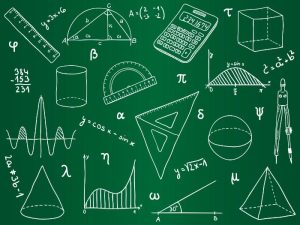## What Does Algebra Mean?

Algebra is one of the most important branches in Mathematics and deals with the study of mathematical symbols, rules, and equations. It is used to solve real-life problems, and it can be useful for analyzing the behavior of various objects.The word algebra is derived from the Arabic term al jebr, which means “reunion of broken parts.” Mathematicians have used the word to describe the process of transforming equations by subtracting like terms from both sides, or passing one term from one side to the other, after changing its sign. The use of the word to denote a distinct subdiscipline of mathematics probably dates from the 16th century, after mathematicians had begun to develop ways to represent and manipulate equations.

### What is the History of Algebra?

The historical development of algebra can be traced from the early era of number systems to the present day. It involves a gradual refinement of a symbolic language suitable for devising and conveying generalized algorithms or step-by-step procedures for solving entire categories of mathematical problems.

Some of the most fundamental concepts in algebra include algebraic expressions and equations, sequence and series, exponents, logarithm, and sets. All of these can be used to solve real-world problems and help students learn how to analyze and solve the complex arithmetic operations in algebra.

### What is the Easiest Way to Learn Algebra?

The easiest way to learn algebra is to start with the basics. The basic arithmetic operations that are taught in algebra include addition, subtraction, multiplication, and division. These are often referred to as the four basic algebraic operations.

### What is the Difference Between Linear and Quadratic Equations?

Linear and quadratic equations are the two most common types of algebraic expressions. They are formed using integer constants, variables, and basic arithmetic operations such as addition, subtraction, and multiplication.

### What Are the Four Basic Rules of Algebra?

The four basic rules of algebra are the commutative rule of addition, commutative rule of multiplication, associative rule of addition, and associative rule of multiplication. These rules are often applied to solve a wide range of problems, and they are also very useful in developing critical thinking skills.

### What Are the Most Popular Topics in Algebra?

The most popular topics in algebra include algebraic expressions and equations, matrices and determinants, sequence and series, and linear functions. These can be used to solve a variety of problems and are an essential part of studying the subject.

### What are the Most Common Problems to Solve With Algebra?

The main purpose of learning algebra is to solve a variety of problems and make sense of data involving two or more variables. These problems can be in the form of simple addition and subtraction problems or more complex ones such as a problem involving a set of prices and a customer’s total.

The most common problem to solve with algebra is the one where an employee has a certain number of boxes of detergent at work and then sells 13 more boxes. The employee would then have 50 boxes left over after the sale. In this case, the employee would have to find a way to reduce the quantity of each box to a specific amount.

In conclusion, algebra is a crucial branch of mathematics that involves the study of mathematical symbols, rules, and equations. It provides a powerful tool for solving real-life problems and analyzing the behavior of various objects. The term “algebra” originates from the Arabic word “al jebr,” which refers to the process of transforming equations. The historical development of algebra has led to the refinement of a symbolic language that enables the formulation and solution of generalized algorithms. Understanding the basics of algebra, including arithmetic operations and the fundamental concepts of algebraic expressions and equations, lays a strong foundation for learning and applying more advanced topics. Linear and quadratic equations are two common types of algebraic expressions, while the four basic rules of algebra, such as the commutative and associative rules, provide a framework for solving problems and developing critical thinking skills. Popular algebra topics include matrices, determinants, sequences, series, and linear functions, which can be applied to solve a variety of problems involving multiple variables. By mastering algebra, individuals gain the ability to make sense of data, solve complex problems, and enhance their analytical and problem-solving skills.